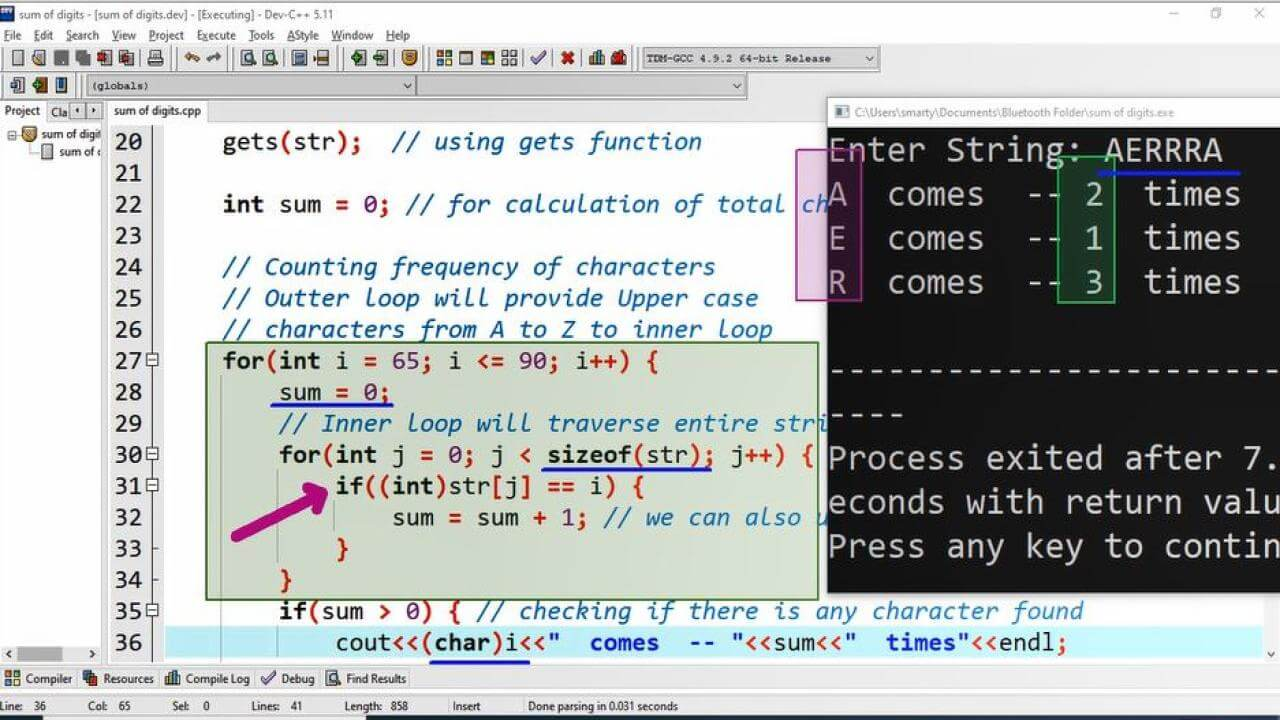# Amount Of Characters In String Program Example using CPP

## Source Code:

#include <iostream>

using namespace std;

/*
String = ABAAC
Here
A = 3 Times
B = 1 Time
C = 1 Time
*/

int main(int argc, char** argv) {

// Initialization of String size 100
char str = "";

// Getting input in string
cout<<"Enter String: ";
gets(str);  // using gets function

int sum = 0; // for calculation of total characters.

// Counting frequency of characters
// Outter loop will provide Upper case
// characters from A to Z to inner loop

for(int i = 65; i <= 90; i++) {
sum = 0;
// Inner loop will traverse entire string
for(int j = 0; j < sizeof(str); j++) {
if((int)str[j] == i) {
sum = sum + 1; // we can also use here sum++
}
}
if(sum > 0) { // checking if there is any character found
cout<<(char)i<<"  comes  -- "<<sum<<"  times"<<endl;
}
}

return 0;
}

## Output:## Working:

In this CPP program we calculate total no of characters from an input string. To implement this algorithm we use here Dev-C++ IDE. First of all we declare a string variable using char data type of size 100. We can also change size of string according to input requirement. We can also declare str variable of string data type intead of char. Then insted of gets() function we will use cin object because gets() function only accept character/char type variables.

After taking input two loops / nested loops are used where outter loop is used to pass alphabets from A to Z to inner loop. The counter variable of outter loop start from 65, which is ASCII number of capital A and run until 90 which is ASCII number of capital Z.

So that in inner loop we are traversing entire string. The inner loop counter variable starts from 0 (which is first index of string) and go to size of string (last character of string) using sizeof operator. In body of inner loop we check if ASCII number of string character which is provided by inner loop is equal to value of outter loop variable said i then this will update value of sum / counter variable.

Finally in body of outter loop each time total amount of characters will print using cout statement. This frequency will print in alphabetic order because our outter loop strats from captial A to capital Z.

For inner as well as outter loop for iterative statement is used.